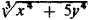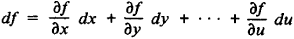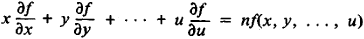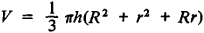Homogeneous Function

Also found in: Wikipedia.

homogeneous function

[‚hä·mə′jē·nē·əs ′fəŋk·shən]
(mathematics)
A real function ƒ(x1, x2, …, xn ) is homogeneous of degree r if ƒ(ax1, ax2, …, axn ) = a rƒ(x1, x2, …, xn ) for every real number a.
McGraw-Hill Dictionary of Scientific & Technical Terms, 6E, Copyright © 2003 by The McGraw-Hill Companies, Inc.
The following article is from The Great Soviet Encyclopedia (1979). It might be outdated or ideologically biased.

Homogeneous Function

a function of one or several variables that satisfies the following condition: when all independent variables of a function are simultaneously multiplied by the same (arbitrary) factor, the value of the function is multiplied by some power of this factor. In algebraic terms, a function f(x, y, …, u) is said to be homogeneous of degree n if for all values of x, y, …, u and for any λ

fx, λy, …, λu) = λnf(x, y, …, u)

For example, the functions x2 – 2y2, (xy – 3z)/(z2 + xy), andare homogeneous of degree 2, –1, and 4/3, respectively. Functions homogeneous of degree n are characterized by Euler’s theorem that asserts that if the differential of each independent variable is replaced with the variable itself in the expression for the complete differentialthen we obtain the function f(x, y, …, u) multiplied by the degree of homogeneity:Homogeneous functions are frequently encountered in geometric formulas. In the equation x = f(a, b, …, l), where a, b, …, l are the lengths of segments expressed in terms of the same unit, f must be a homogeneous function (of degree 1, 2, or 3, depending on whether x signifies length, area, or volume). For example, in the formula for the volume of a truncated coneV is a homogeneous function of degree 3 in h, R, and r.

References in periodicals archive ?
Let [OMEGA] [member of] [Lip.sub.[beta]]([R.sup.n]) for 0 < [beta] [less than or equal to] 1 be a homogeneous function of degree zero and
Suppose V : [R.sup.n] [right arrow] R is a homogeneous function of degree t with respect to the dilation weight [DELTA].
where a'([xi]) is homogeneous function of degree zero in [xi].
Therefore it is enough to prove the statement for a homogeneous function f.
Suppose V: [R.sup.n] [right arrow] R is a homogeneous function of degree [tau] with respect to the dilation weight [DELTA].
In , the authors introduced the homogeneous function with two parameters r and s by,
Zhen-Hang Yang, defined and studied the monotonicity of Homogeneous function [{[[f([a.sup.p], [b.sup.p])]/[f([a.sup.q], [b.sup.q])]]}.sup.[1/[p - q]]] and established inequalities involving various means and some mean identities.
where [([G.sup.-]).sup.ml] is the element of the matrix [G.sup.-] and F([omega], [xi]) is a homogeneous function of degree -2, because the elements of the matrices [U.sup.[+ or -]] and [R.sup.[+ or -]] are homogeneous functions of degree zero, while the elements of the matrices [S.sup.[+ or -]] are homogeneous functions of degree -2.
In general, such a linearly homogeneous function F(x) is an FFF only if the true function V(x) is also homogeneous of degree one.
Had he realized it, Wicksteed could have deduced adding-up directly from Leonhard Euler's famous mathematical theorem on homogeneous functions. That theorem says that any linear homogeneous function can be written as the sum of its first partial derivatives each multiplied by the associated independent variable.
which is clearly the composition of the map w [??] ([absolute value of ([w.sub.0])], [absolute value of ([w.sub.(1)])], ..., [absolute value of ([w.sub.(l)])] with a homogeneous function of degree 0.
A homogeneous function of degree one is called linearly homogeneous.

Site: Follow: Share:
Open / Close